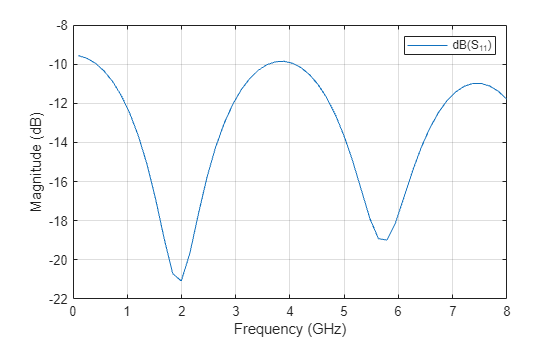# Design of Quarter-Wave Transformer for Impedance Matching Applications

This example shows how to design the Quarter-wave transformer for impedance matching applications by using the `pcbComponent, microstripLine, `and` traceRectangular `object in the RF PCB Toolbox.

Quarter-wave transformer is a simple and useful circuit for matching the real impedance of a terminating load (${Z}_{L}$) to the characteristic impedance of the feeding transmission line (${Z}_{0}$) as depicted in the given figure. The characteristic impedance of the quarter-wave transformer is calculated as ${\mathit{Z}}_{1}=\sqrt{\left({\mathit{Z}}_{0}{\text{\hspace{0.17em}}\mathit{Z}}_{\mathit{L}}\right)}\text{\hspace{0.17em}}$. This example is to design a single section quarter-wave transformer to match the$100\text{\hspace{0.17em}}\Omega$ load to a $50\text{\hspace{0.17em}}\Omega \text{\hspace{0.17em}}$transmission line at an operating frequency of $2\text{\hspace{0.17em}}\mathrm{GHz}$. The calculated characteristic impedance of the quarter-wave transformer ${\mathit{Z}}_{1}$is $70.71\text{\hspace{0.17em}}\Omega$.### Design of Single Section Quarter-Wave Transformer

Use the `design` function on the `microstripLine` object to create the $50\text{\hspace{0.17em}}\Omega \text{\hspace{0.17em}}$input transmission line and$70.71\text{\hspace{0.17em}}\Omega \text{\hspace{0.17em}}$quarter-wave transformer's `Length` and `Width `dimension for the operating frequency of $2\text{\hspace{0.17em}}\mathrm{GHz}$. The default substrate for `microstripLine` is Teflon with thickness of $1.6\text{\hspace{0.17em}}\mathrm{mm}$.

```freq = 2e9; m50 = design(microstripLine,freq,Z0=50,LineLength=0.05); % input transmission line m70 = design(microstripLine,freq,Z0=70.71,LineLength=0.25); % section 1```

Use the `traceRectangular` object to create the groundplane, input transmission line, and quarter-wave transmission line shapes.

```% ground plane dimension gndL = 2*m50.Length+m70.Length; gndW = 5*m50.Width; ground = traceRectangular("Length",gndL,"Width",gndW); % input transmission line Z0 = traceRectangular("Length",m50.Length,"Width",m50.Width,... "Center",[-gndL/2+m50.Length/2 0]); % First section Quarter-wave transformer Z1 = traceRectangular("Length",m70.Length,"Width",m70.Width,"Center",... [-gndL/2+m50.Length+m70.Length/2 0]); qline = Z0 + Z1;```

Use the `pcbComponent `to create the quarter-wave transformer and use the `lumpedElement` for the terminating load of $100\text{\hspace{0.17em}}\Omega$ and place it at the end of the quarter-wave transformer and use a via to connect the load to ground.

```pcb =pcbComponent; pcb.BoardShape = ground; pcb.BoardThickness = m50.Height; pcb.Layers = {qline,m50.Substrate,ground}; pcb.FeedDiameter = m50.Width/2; pcb.FeedLocations = [-gndL/2 0 1 3]; pcb.ViaLocations = [-gndL/2+m50.Length+m70.Length,0,1,3]; pcb.ViaDiameter = m70.Width/2; % Load ZL = lumpedElement; ZL.Impedance = 100; ZL.Location = [-gndL/2+m50.Length+m70.Length,0,pcb.BoardThickness]; pcb.Load = ZL; % show the single section quarter-wave transformer figure;show(pcb)```Use the `sparameters` function to calculate the S parameters and plot it using the `rfplot` function.

```sparams = sparameters(pcb,linspace(100e6,8e9,51)); figure; rfplot(sparams)```It is observed from the ${\mathit{S}}_{11}$ values that the impedance is perfectly matched at the desired frequency of $2\text{\hspace{0.17em}}\mathrm{GHz}$ where the value of magnitude is less than $-22\text{\hspace{0.17em}}\mathrm{dB}$ with the bandwith of $2800\text{\hspace{0.17em}}\mathrm{MHz}$. Matching alsol occurs at frequencies where the ${\mathit{Z}}_{1}$has a length of $\left(2\mathit{n}+1\right){\lambda }_{0}}{4},\mathit{n}=0,1,2,3...$

### Design of Multisection Quarter-Wave Transformer

In general, the single section transformer may be sufficient for narrow band impedance match. This transformer can be extended to multisection in a methodical manner to yield optimum matching characteristics over a wider bandwidth . The following example is for the design of three section Chebyshev matching transformer to match a $100\text{\hspace{0.17em}}\Omega$ load to a $50\text{\hspace{0.17em}}\Omega \text{\hspace{0.17em}}$ with ripple level = $0.05$. Each section's characteristic impedances are computed using the formulas given in  and the values are ${\mathit{Z}}_{1}=57.5\Omega ,{\text{\hspace{0.17em}}\mathit{Z}}_{2}=70.7\Omega$ and ${\mathit{Z}}_{3}=\text{\hspace{0.17em}}87\Omega$. The three section Chebyshev matching transformer is designed by using same steps described in the design of single section quarter-wave transformer.

```m50 = design(microstripLine,freq,"Z0",50,"LineLength",0.05); % input transmission line m57 = design(microstripLine,freq,"Z0",57.5,"LineLength",0.25); % section 1 m70 = design(microstripLine,freq,"Z0",70.7,"LineLength",0.25); % section 2 m87 = design(microstripLine,freq,"Z0",87,"LineLength",0.25); % section 3 % ground plane dimension gndL = 2*m50.Length+m57.Length+m70.Length+m87.Length; gndW = 5*m50.Width; ground = traceRectangular("Length",gndL,"Width",gndW); % inpt transmission line Z0 = traceRectangular("Length",m50.Length,"Width",m50.Width,... "Center",[-gndL/2+m50.Length/2 0]); % First section Quarter-wave transformer Z1 = traceRectangular("Length",m57.Length,"Width",m57.Width,"Center",... [-gndL/2+m50.Length+m57.Length/2 0]); % Second section Quarter-wave transformer Z2 = traceRectangular("Length",m70.Length,"Width",m70.Width,"Center",... [-gndL/2+m50.Length+m57.Length+m70.Length/2 0]); % Third section Quarter-wave transformer Z3 = traceRectangular("Length",m87.Length,"Width",m87.Width,"Center",... [-gndL/2+m50.Length+m57.Length+m70.Length+m87.Length/2 0]); qline = Z0+Z1+Z2+Z3; % create pcbComponent pcb =pcbComponent; pcb.BoardShape = ground; pcb.BoardThickness = m50.Height; pcb.Layers ={qline,m50.Substrate,ground}; pcb.FeedDiameter = m50.Width/2; pcb.FeedLocations = [-gndL/2 0 1 3]; pcb.ViaLocations = [-gndL/2+m50.Length+m57.Length+m70.Length+m87.Length,0,1,3]; pcb.ViaDiameter = m87.Width/2; % Load ZL = lumpedElement; ZL.Impedance = 100; ZL.Location = [-gndL/2+m50.Length+m57.Length+m70.Length+m87.Length,0,pcb.BoardThickness]; pcb.Load = ZL; % show the three section quarter-wave transformer figure;show(pcb)``````% Analysis sparams3 = sparameters(pcb,linspace(100e6,8e9,51)); figure; rfplot(sparams3)```#### Comparison of S parameters

```figure; rfplot(sparams,'-.') hold on rfplot(sparams3) legend('dB(S_{11}) for N=1','dB(S_{11}) for N=3','Location','northeast')```From the comparison the s-parameters plot for single section and three section quarter-wave transformers designed at $2\text{\hspace{0.17em}}\mathrm{GHz}$, it is observed that the bandwidth achieved for three section quarter-wave transformer is $7750\text{\hspace{0.17em}}\mathrm{MHz}\text{\hspace{0.17em}}$with improved impedance matching characteristics.Therefore, it is evident that wider bandwidth is achieved for quarter-wave transformers with multiple sections.

### References

 David M. Pozar, Microwave Engineering, pp. 246-261, ${4}^{\mathrm{th}}$ Edition, John Wiley & Sons, 2012.